# heatmap

Create heatmap chart

## Syntax

``heatmap(tbl,xvar,yvar)``
``heatmap(tbl,xvar,yvar,'ColorVariable',cvar)``
``heatmap(cdata)``
``heatmap(xvalues,yvalues,cdata)``
``heatmap(___,Name,Value)``
``heatmap(parent,___)``
``h = heatmap(___)``

## Description

example

````heatmap(tbl,xvar,yvar)` creates a heatmap from the table `tbl`. The `xvar` input indicates the table variable to display along the x-axis. The `yvar` input indicates the table variable to display along the y-axis. The default colors are based on a count aggregation, which totals the number of times each pair of x and y values appears together in the table.```

example

````heatmap(tbl,xvar,yvar,'ColorVariable',cvar)` uses the table variable specified by `cvar` to calculate the color data. The default calculation method is a mean aggregation.```

example

````heatmap(cdata)` creates a heatmap from matrix `cdata`. The heatmap has one cell for each value in `cdata`.```

example

````heatmap(xvalues,yvalues,cdata)` specifies the labels for the values that appear along the x-axis and y-axis.```

example

````heatmap(___,Name,Value)` specifies additional options for the heatmap using one or more name-value pair arguments. Specify the options after all other input arguments. For a list of properties, see HeatmapChart Properties.```
````heatmap(parent,___)` creates the heatmap in the figure, panel, or tab specified by `parent`.```
````h = heatmap(___)` returns the `HeatmapChart` object. Use `h` to modify properties of the chart after creating it. For a list of properties, see HeatmapChart Properties.```

## Examples

collapse all

Create a heatmap from a table of data for medical patients.

Load the `patients` data set and create a table from a subset of the variables loaded into the workspace. Then create a heatmap that counts the total number of patients with the same set of `Smoker` and `SelfAssessedHealthStatus` values.

```load patients tbl = table(LastName,Age,Gender,SelfAssessedHealthStatus,... Smoker,Weight,Location); h = heatmap(tbl,'Smoker','SelfAssessedHealthStatus');```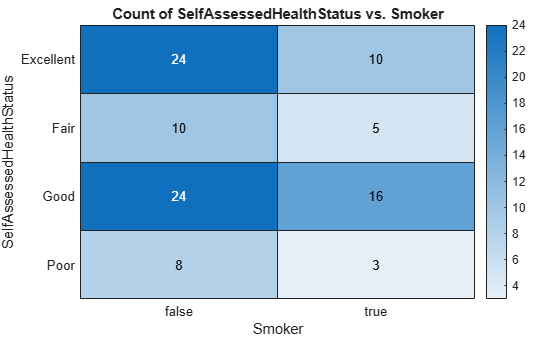Create a heatmap and reorder the labels along the y-axis.

Load the `patients` data set and create a heatmap from the data. Assign the `HeatmapChart` object to the variable `h`.

```load patients tbl = table(LastName,Age,Gender,SelfAssessedHealthStatus,... Smoker,Weight,Location); h = heatmap(tbl,'Smoker','SelfAssessedHealthStatus');```Reorder the labels along the y-axis.

`h.YDisplayData = {'Excellent','Good','Fair','Poor'};`Alternatively, you can reorder the labels by changing the data to categorical data and then reordering the categories using the `reordercats` function. Similarly, you can add, remove, or rename the heatmap labels using the `addcats`, `removecats`, or `renamecats` functions, respectively.

Create a heatmap and specify the table variable to use when determining the heatmap cell colors.

Load the `patients` data set and create a heatmap from the data. Color each cell using the average age of patients with a particular pair of `Smoker` and `SelfAssessedHealthStatus` values by setting the `ColorVariable` option to `'Age'`.

```load patients tbl = table(LastName,Age,Gender,SelfAssessedHealthStatus,... Smoker,Weight,Location); h = heatmap(tbl,'Smoker','SelfAssessedHealthStatus','ColorVariable','Age');```Create a heatmap and specify the table variable and calculation method to use when determining the heatmap cell colors.

Load the patients data set and create a heatmap from the data. Color each cell using the median age of patients with a particular pair of `Smoker` and `SelfAssessedHealthStatus` values. Specify the `ColorVariable` option as `'Age'` and the `ColorMethod` option as `'median'`.

```load patients tbl = table(LastName,Age,Gender,SelfAssessedHealthStatus,... Smoker,Weight,Location); h = heatmap(tbl,'Smoker','SelfAssessedHealthStatus','ColorVariable','Age','ColorMethod','median');```Create a matrix of data. Then create a heatmap of the matrix values. The default labels along the x-axis and y-axis appear as 1, 2, 3, and so on.

```cdata = [45 60 32; 43 54 76; 32 94 68; 23 95 58]; h = heatmap(cdata);```Create a matrix of data. Then create a heatmap of the matrix values. Use custom labels along the x-axis and y-axis by specifying the first two input arguments as the labels you want. Specify the title and axis labels by setting properties of the `HeatmapChart` object.

```cdata = [45 60 32; 43 54 76; 32 94 68; 23 95 58]; xvalues = {'Small','Medium','Large'}; yvalues = {'Green','Red','Blue','Gray'}; h = heatmap(xvalues,yvalues,cdata); h.Title = 'T-Shirt Orders'; h.XLabel = 'Sizes'; h.YLabel = 'Colors';```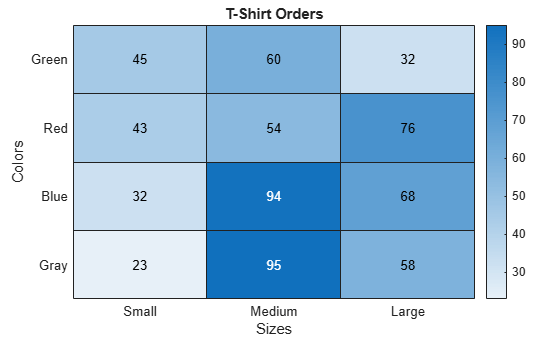Create a heatmap and normalize the colors along each column or row by setting the `ColorScaling` property.

Read the sample file `outages.csv` into a table. The sample file contains data representing electric utility outages in the Unites States. The table contains six columns: `Region`, `OutageTime`, `Loss`, `Customers`, `RestorationTime`, and `Cause`. Display the first five rows of each column.

```T = readtable('outages.csv'); T(1:5,:)```
```ans=5×6 table Region OutageTime Loss Customers RestorationTime Cause _____________ ________________ ______ __________ ________________ ___________________ {'SouthWest'} 2002-02-01 12:18 458.98 1.8202e+06 2002-02-07 16:50 {'winter storm' } {'SouthEast'} 2003-01-23 00:49 530.14 2.1204e+05 NaT {'winter storm' } {'SouthEast'} 2003-02-07 21:15 289.4 1.4294e+05 2003-02-17 08:14 {'winter storm' } {'West' } 2004-04-06 05:44 434.81 3.4037e+05 2004-04-06 06:10 {'equipment fault'} {'MidWest' } 2002-03-16 06:18 186.44 2.1275e+05 2002-03-18 23:23 {'severe storm' } ```

Create a heatmap that shows the different regions along the x-axis and the different outage causes along the y-axis. In each cell, show how many times each region experienced a power outage due to a particular cause.

`h = heatmap(T,'Region','Cause');`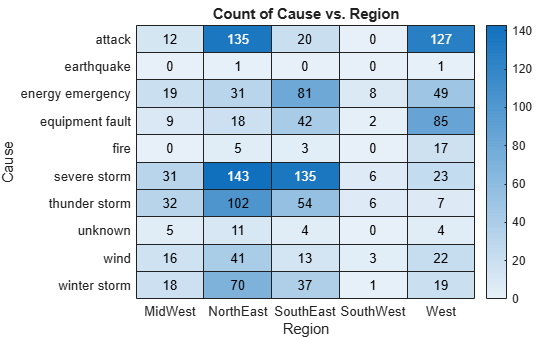Normalize the colors along each column. The smallest value in each column maps to the first color in the colormap and the largest value maps to the last color. The last color indicates the cause that caused the most power outages for each region.

`h.ColorScaling = 'scaledcolumns';`Normalize the colors along each row instead. The smallest value in each row maps to the first color in the colormap and the largest value maps to the last color. The last color indicates the region that experienced the most power outages due to each cause.

`h.ColorScaling = 'scaledrows';`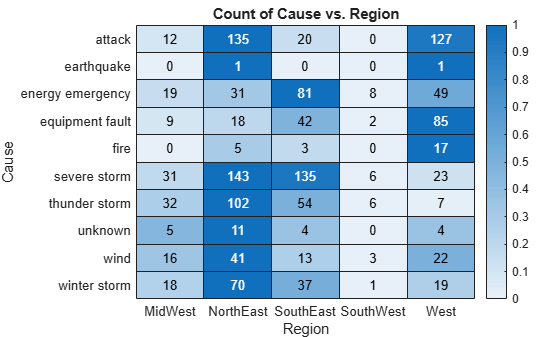Create a heatmap and color the cells using data computed with a custom aggregation method. Use the `accumarray` function to compute the color data.

Read the sample file `Temperature.csv` into a table. The file contains three columns: `Month`, `Year`, and `TemperatureF`.

`tbl = readtable('TemperatureData.csv');`

Create categorical arrays from the `Month` and `Year` columns of the table. Then determine the unique months and years to use as labels along the x-axis and y-axis.

```months = categorical(tbl.Month); years = categorical(tbl.Year); xlabels = categories(months); ylabels = categories(years);```

Determine the final size of the resulting color data based on the number of unique months and years.

```nummonths = numel(xlabels); numyears = numel(ylabels);```

Convert the categorical `months` and `years` arrays into numeric indices to use with the `accumarray` function. Compute the color data as the maximum temperature for each month and year combination using the `accumarray` function. Use `NaN` for missing month and year combinations.

```x = double(months); y = double(years); temps = tbl.TemperatureF; cdata = accumarray([y,x],temps,[numyears,nummonths],@max,NaN);```

Create the heatmap. Label the x-axis and y-axis with the months and years, respectively. Color the heatmap cells using the computed matrix data.

`h = heatmap(xlabels,ylabels,cdata);`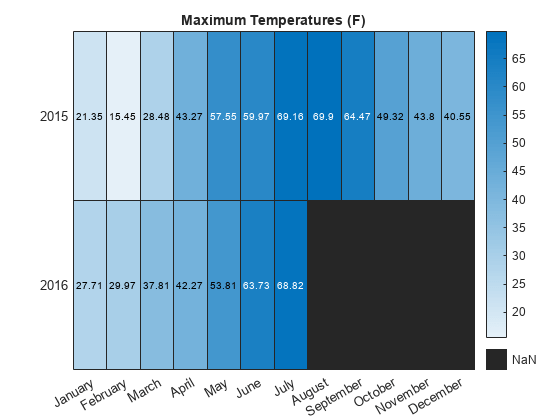Note: You can use the `reordercats` function for categorical arrays to reorder the axis labels.

## Input Arguments

collapse all

Source table, specified as a table or timetable.

You can create a table from workspace variables using the `table` function, or you can import data as a table using the `readtable` function. You can create a timetable from workspace variables using the `timetable` function.

The `SourceTable` property of the `HeatmapChart` object stores the source table.

Table variable for x-axis, specified in one of these forms:

• Character vector or string scalar indicating one of the variable names. For example, `heatmap(tbl,'HealthStatus','Gender')` selects the variable named `'HealthStatus'` for the x-axis.

• Numeric scalar indicating the table variable index. For example, `heatmap(tbl,2,3)` selects the second variable in the table for the x-axis.

• Logical vector containing one `true` element.

The values associated with your table variable must be grouped into a finite set of discrete categories that the `categorical` function accepts. If the values are not grouped into a finite set of categories, use the `discretize` function to group them.

The labels that appear along the x-axis are in alphabetical order. You can customize the labels using categorical arrays. For an example, see Create Heatmap from Tabular Data.

The `XVariable` property of the `HeatmapChart` object stores the selected variable.

Table variable for y-axis, specified in one of these forms:

• Character vector or string scalar of one of the variable names. For example, `heatmap(tbl,'HealthStatus','Gender')` selects the variable named `'HealthStatus'` for the y-axis.

• Numeric scalar indicating the table variable index. For example, `heatmap(tbl,2,3)` selects the third variable in the table for the y-axis.

• Logical vector containing one `true` element.

The values associated with your table variable must be grouped into a finite set of discrete categories that the `categorical` function accepts. If the values are not grouped into a finite set of categories, use the `discretize` function to group them.

The labels that appear along the y-axis are in alphabetical order. You can customize the labels using categorical arrays. For an example, see Create Heatmap from Tabular Data.

The `YVariable` property of the `HeatmapChart` object stores the selected variable.

Table variable for color data, specified in one of these forms:

• A character vector or string scalar of one of the variable names. For example, `heatmap(__,'ColorVariable','HealthStatus')` selects the variable named `'HealthStatus'` for the y-axis.

• A numeric scalar indicating the table variable index. For example, `heatmap(__,'ColorVariable',1)` selects the third variable in the table for the y-axis.

• A logical vector containing one `true` element.

The values associated with your table variable must be of a numeric type or `logical`.

The property value is empty `[]` when using matrix data. The `ColorVariable` property of the `HeatmapChart` object stores the selected variable. The `ColorData` property populates with data based on the selected variable.

By default, `heatmap` calculates the color data based on a mean aggregation. To change the calculation method, set the `ColorMethod` property.

Color data for the heatmap cells, specified as a matrix.

The `ColorData` property of the `HeatmapChart` object stores the values.

Example: `[40 24 68; 68 37 58; 49 23 46]`

Values appearing along the x-axis, specified as a categorical array, string array, numeric array, or cell array of character vectors.

Example: `{'small','medium','large'}`

Example: `categorical({'small','medium','large'})`

Values appearing along y-axis, specified as a categorical array, string array, numeric array, or cell array of character vectors.

Example: `{'small','medium','large'}`

Example: `categorical({'small','medium','large'})`

Parent container, specified as a `Figure`, `Panel`, `Tab`, `TiledChartLayout`, or `GridLayout` object.

### Name-Value Pair Arguments

Specify optional comma-separated pairs of `Name,Value` arguments. `Name` is the argument name and `Value` is the corresponding value. `Name` must appear inside quotes. You can specify several name and value pair arguments in any order as `Name1,Value1,...,NameN,ValueN`.

Example: `heatmap(tbl,xvar,yvar,'ColorVariable','Temperatures','ColorMethod','median')`

Note

The properties listed here are only a subset. For a complete list, see HeatmapChart Properties.

Chart title, specified as a character vector, cell array of character vectors, string array, or categorical array.

Example: ```h = heatmap(rand(10,10),'Title','My Title Text')```

Example: `h.Title = 'My Title Text';`

To create a multiline title, specify a cell array of character vectors or a string array. Each element in the array corresponds to a line of text.

Example: `h.Title = {'My','Title'};`

If you specify the title as a categorical array, MATLAB® uses the values in the array, not the categories.

If you create the chart using tabular data, the default chart has an autogenerated title. If you do not want a title, specify `''`.

By default, heatmaps support a subset of TeX markup for the text you specify. Use TeX markup to add superscripts and subscripts, modify the font type and color, and include special characters in the text. If you want a TeX markup character in regular text, such as an underscore (_), then insert a backslash (\) before the character you want to include. The backslash is the TeX escape character. For more information, see the Interpreter property of the text object.

Method to calculate the color data values (stored in `ColorData`), specified as `'count'`, `'mean'`, `'median'`, `'sum'`, `'max'`, `'min'`, or `'none'`.

If you do not want to use a third variable from the table for the color data, then specify the method as `'count'`. When the method is `'count'`, the heatmap does not use the `ColorVariable` property and instead counts the number of times each pair of x and y values appears in the source table. This value is the default value when you are using tabular data and do not specify the `ColorVariable` parameter.

If you want to use a third variable from the table for the color data, then set the `ColorVariable` property to the variable you want and specify the `ColorMethod` property as one of the methods listed in this table. For each pair of x and y values, the methods use the corresponding values in the `ColorVariable` column of the source table to calculate the data.

MethodDescription
`'mean'`Calculate the average value. This value is the default value when you specify the `ColorVariable` property.
`'median'`Calculate the median value.
`'sum'`Sum the values.
`'max'`Use the maximum value.
`'min'`Use the minimum value.
`'none'`Use the value exactly. The table cannot contain more than one instance of each pair of x and y values. This value is the default value when using matrix data.

If you want to compute your own matrix of aggregated data, use the `accumarray` function. Specify the matrix as input to the `heatmap` function.

Example: `h = heatmap(__,'ColorMethod','median')`

Example: `h.ColorMethod = 'median'`

## Output Arguments

collapse all

`HeatmapChart` object, which is a standalone visualization. Use `h` to set properties on the heatmap chart after creating it.

collapse all

### Standalone Visualization

A standalone visualization is a chart designed for a special purpose that works independently from other charts. Unlike other charts such as `plot` and `surf`, a standalone visualization has a preconfigured axes object built into it, and some customizations are not available. A standalone visualization also has these characteristics:

• It cannot be combined with other graphics elements, such as lines, patches, or surfaces. Thus, the `hold` command is not supported.

• The `gca` function can return the chart object as the current axes.

• You can pass the chart object to many MATLAB functions that accept an axes object as an input argument. For example, you can pass the chart object to the `title` function.

## Tips

• To interactively explore the data in your heatmap, use these options.

• Zoom — Use the scroll wheel or the + and - keys to zoom.

• Pan — Click and drag the heatmap or use the arrow keys to pan across the rows or columns.

• Data tips — Hover over the heatmap to display a data tip.

• Rearrange rows and columns — Click and drag a row or column label to move it to a different position.

• Sort values — Click the icon that appears when you hover over the row or column label. Click once to sort the values in ascending order, twice to sort the values in descending order, and a third time to reset the order.

• If you create a heatmap chart from a table, then you can customize its data tips.

• To add or remove a row from the data tip, right-click anywhere on the chart and point to Modify Data Tips. Then, select or deselect a variable.

• To add or remove multiple rows, right-click on the chart, point to Modify Data Tips, and select More. Then, add variables by clicking or remove them by clicking .

## Compatibility Considerations

expand all

Behavior changed in R2019a

### Topics

Introduced in R2017a